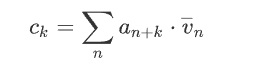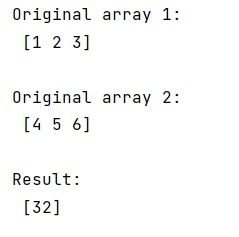# Compute cross-correlation of two given NumPy arrays

Learn, how can we compute cross-correlation of two given NumPy arrays?
Submitted by Pranit Sharma, on March 20, 2023

## Cross correlate 1d arrays

Correlation refers to a process for establishing the relationships between two variables. This function computes the correlation as generally defined in signal-processing texts:Suppose that we are given two 1D arrays, and we need to find the cross-correlation between them.

To compute cross-correlation of two arrays, we can simply use the numpy.correlate2d() method.

Parameter(s)

• a, v: input arrays
• mode: it is a string which represent the mode of output. There are different modes like full, valid, and same.

Let us understand with the help of an example,

## Python code to compute cross-correlation of two given NumPy arrays

```# Import numpy
import numpy as np

# Creating two numpy arrays
arr1 = np.array([1,2,3])
arr2 = np.array([4,5,6])

# Display original arrays
print("Original array 1:\n",arr1,"\n")
print("Original array 2:\n",arr2,"\n")

# Finding cross correlation
res = np.correlate(arr1,arr2)

# Display result
print("Result:\n",res,"\n")
```

### Output